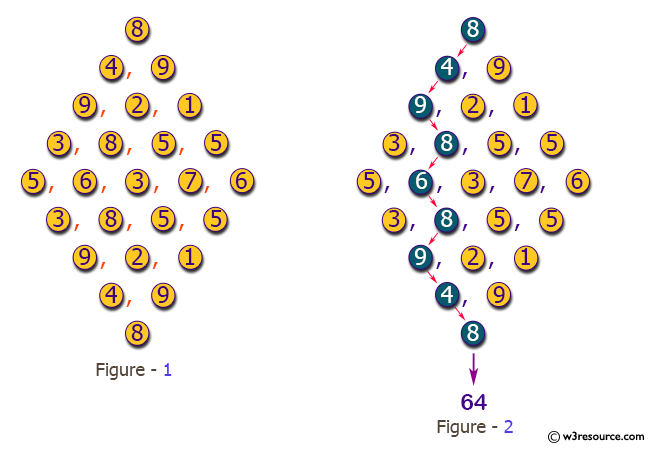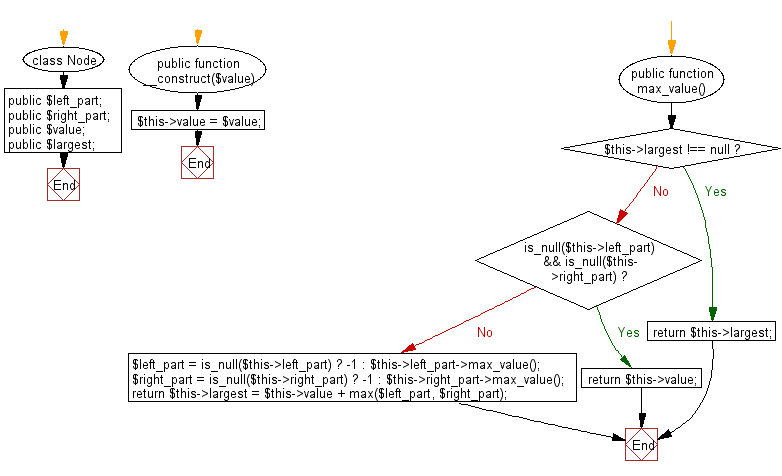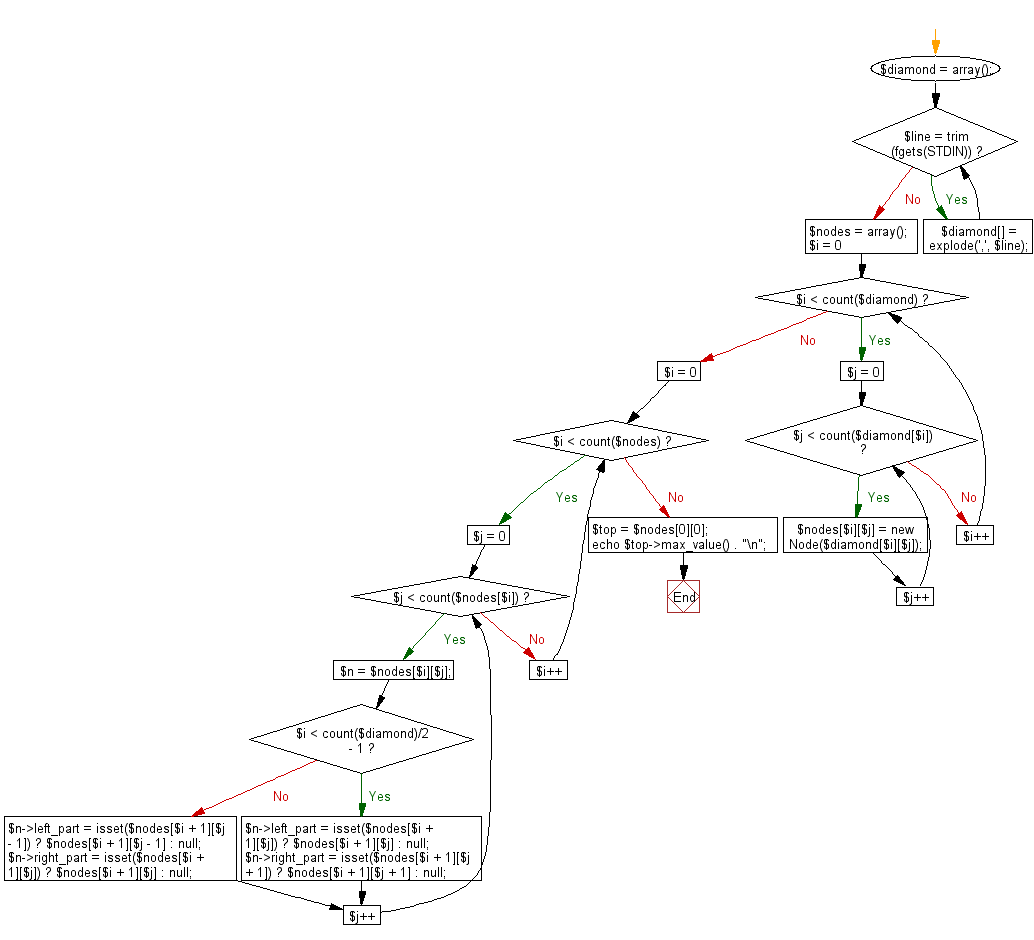﻿ PHP Exercise: Compute the maximum value of the sum of the passing integers - w3resource

# PHP Exercises: Compute the maximum value of the sum of the passing integers

## PHP: Exercise-75 with Solution

Arrange integers (0 to 99) as narrow hilltop, as illustrated in Figure 1. Reading such data representing huge, when starting from the top and proceeding according to the next rule to the bottom. Write a PHP program that compute the maximum value of the sum of the passing integers.

Pictorial Presentation:Input: A series of integers separated by commas are given in diamonds. No spaces are included in each line. The input example corresponds to Figure 1. The number of lines of data is less than 100 lines.

Sample Output:
The maximum value of the sum of integers passing according to the rule on one line.

Sample Solution: -

PHP Code:

``````<?php
class Node {
public \$left_part;
public \$right_part;
public \$value;
public \$largest;

public function __construct(\$value) {
\$this->value = \$value;
}

public function max_value() {
if (\$this->largest !== null) {
return \$this->largest;
}
if (is_null(\$this->left_part) && is_null(\$this->right_part)) {
return \$this->value;
}
\$left_part  = is_null(\$this->left_part)  ? -1 : \$this->left_part->max_value();
\$right_part = is_null(\$this->right_part) ? -1 : \$this->right_part->max_value();

return \$this->largest = \$this->value + max(\$left_part, \$right_part);
}
}

\$diamond = array();

while (\$line = trim(fgets(STDIN))) {
\$diamond[] = explode(',', \$line);
}

\$nodes = array();
for (\$i = 0; \$i < count(\$diamond); \$i++) {
for (\$j = 0; \$j < count(\$diamond[\$i]); \$j++) {
\$nodes[\$i][\$j] = new Node(\$diamond[\$i][\$j]);
}
}

for (\$i = 0; \$i < count(\$nodes); \$i++) {
for (\$j = 0; \$j < count(\$nodes[\$i]); \$j++) {
\$n = \$nodes[\$i][\$j];
if (\$i < count(\$diamond)/2 - 1) {
\$n->left_part  = isset(\$nodes[\$i + 1][\$j])     ? \$nodes[\$i + 1][\$j]     : null;
\$n->right_part = isset(\$nodes[\$i + 1][\$j + 1]) ? \$nodes[\$i + 1][\$j + 1] : null;
} else {
\$n->left_part  = isset(\$nodes[\$i + 1][\$j - 1]) ? \$nodes[\$i + 1][\$j - 1] : null;
\$n->right_part = isset(\$nodes[\$i + 1][\$j])     ? \$nodes[\$i + 1][\$j]     : null;
}
}
}

\$top = \$nodes;

echo \$top->max_value() . "\n";

?>
```
```

Sample Input:
8
4, 9
9, 2, 1
3, 8, 5, 5
5, 6, 3, 7, 6
3, 8, 5, 5
9, 2, 1
4, 9
8

Sample Output:

`64`

Flowchart:Flowchart:PHP Code Editor:

Have another way to solve this solution? Contribute your code (and comments) through Disqus.

What is the difficulty level of this exercise?

Test your Programming skills with w3resource's quiz.

﻿

## PHP: Tips of the Day

Returns all elements in an array except for the first one

Example:

```<?php
function tips_tail(\$items)
{
return count(\$items) > 1 ? array_slice(\$items, 1) : \$items;
}

print_r(tips_tail([1, 5, 7]));
?>
```

Output:

```Array
(
 => 5
 => 7
)
```